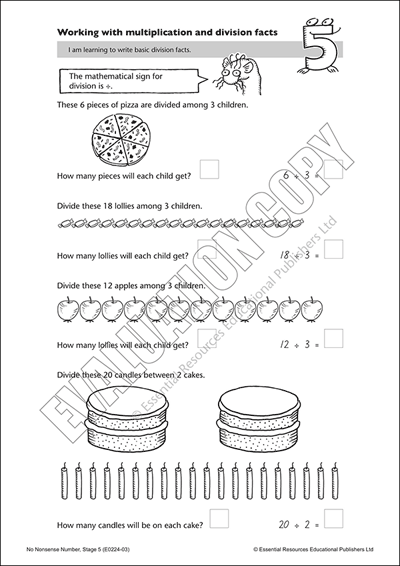# Learning to write multiplication and division facts

Code: E0224-03The variety of multiplication and division tasks in this unit reinforce these concepts. In the first activity students complete basic division equations by grouping the corresponding images. The second task requires students to write the division sum based on the multiplication fact in each picture. Following this there are two pages of equations in which the divisor or multiplier is written inside a hippo, making these number problems look more engaging. In the final activity, students write the multiplication or division fact that goes with the one already written on each leaf on the tree.

Subjects: | Resource Types: | Pages: 5 | Years: 3-4

 or more each